# Geometric shape area calculator

If your roof is a bit more complex, you can try to calculate the surface area of your roof by splitting it into geometrical shapes which you calculate individually and then you can sum them to get the total roof surface area.
Here are some basic shapes calculators for this purpose.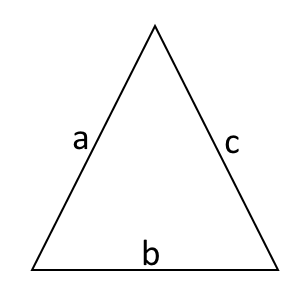*All fields are required:
a
b
c
*For decimal dimensions, use the dot notation! (.)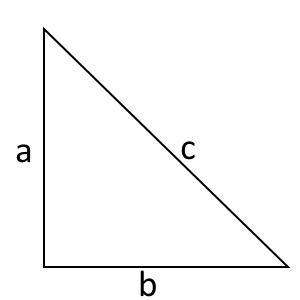*Only 2 dimensions (any) are required:
a
b
c
*For decimal dimensions, use the dot notation! (.)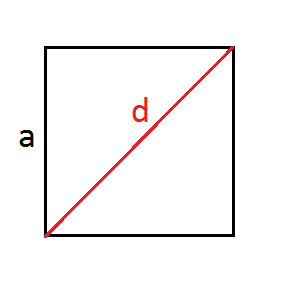*Only one field is required (any):
a
d
*For decimal dimensions, use the dot notation! (.)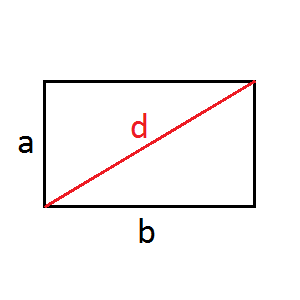a
b
d
*For decimal dimensions, use the dot notation! (.)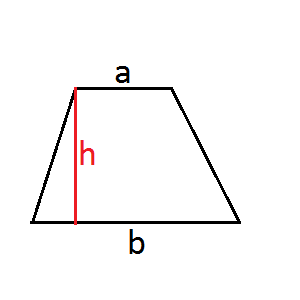*All fields are required:
a
b
h
*For decimal dimensions, use the dot notation! (.)